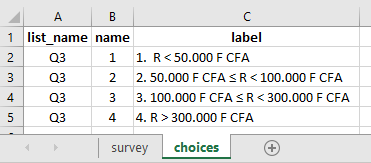# Automatic selection of one answer based on another

Hello. Is it possible to auto-select a single-choice answer based on the answer to the previous question?
I have inserted a numeric field to allow the farmer to give with exactitude the income obtained during the agricultural campaign.
The next question will be to estimate an income obtained in a price range, in case the latter does not have with precision, or does not remember exactly what he will have earned.
The problem is as follows:
1- When the farmer gives with precision the amount he has won, then the following question automatically places or predefined this amount in the price range corresponding to what he obtained.
2- If this is not the case, then we move on to the next question which will consist in asking him in this price interval for the estimated amount of his gain.
anM8Y3vqJ2YnUs22ZkKwFP.xlsx (9.9 KB)

So you mean, if a user enters an integer in Q1, the system should automatically enter the specific range in Q3?

Excuse me, I did not clarify something. The value in Q1 is the weight of the output.
I insert a hidden value formula so that it automatically calculates the gain obtained is Q1 * 750 F CFA which gives the amount obtained.
Then the correspondence of this amount calculated on the basis of the weight and the price per kilogram (750) to be able to be located automatically in one of the options which is in question 3.
I tried this formula but no result ink:
if (\$ {calculation} <50000, ‘1’, if ((\$ {calculation> 50000 and (\$ {calculation} <100000, ‘2’, if (\$ {calculation}> 100000 and (\$ {calculation} <300000 , ‘3’, if (\$ {Revenue_Cacao_2019-2020}> 400000, ‘4’ "))))

So what you are trying to solve is that if Q1 has 100, the obtained score is `75000`. Now you wish to auto select `option 2` in `Q3`?

yes exactly.

In this case you could do it as outlined in the image shared below:

#### In the choices tab of your xlsform:#### Reference xlsform:

Prefill (if-statement).xlsx (11.5 KB)

2 Likes

Thanks Kal.